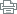skip navigation

This page is designed for modern browsers. You will have a better experience with a better browser.# Associate in Science in Mathematics for Transfer

## Victor Valley College

### 1. Statement of Program Goals and Objectives

This degree is designed for students who wish to transfer to a California State University to complete a bachelor's degree in mathematics or a related field. All of the courses in the degree plan align with the Transfer Model Curriculum (TMC) for Mathematics and will prepare students for a baccalaureate degree in mathematics or a related field.

The goal of the Mathematics for Transfer major is to produce graduates who successfully transfer to major universities to major in mathematics. The outcome to be measured will be the number of transfer students produced annually with the AS-T in Mathematics.

The VVC mathematics department has the following Program Level Outcomes (PLOs):

1. define arithmetic, algebraic, geometric, spatial, and statistical concepts.
2. calculate arithmetic, algebraic, geometric, spatial, and statistical quantities using appropriate technology.
3. estimate arithmetic, algebraic, geometric, spatial, and statistical solutions.
4. solve arithmetic, algebraic, geometric, spatial, and statistical expressions, equations, functions, and problems using appropriate technology.
5. represent mathematical information numerically, symbolically, graphically, verbally, and visually using appropriate technology.
6. develop mathematical and statistical models such as formulas, functions, graphs, tables, and schematics using appropriate technology.
7. interpret mathematical and statistical models such as formulas, functions, graphs, tables, and schematics, drawing conclusions and making inferences based on those models.
8. communicate mathematical thoughts and ideas clearly and concisely to others in the oral and written form.

### 2. Catalog Description

The role of mathematics is vital and growing, providing solutions to problems in a wide range of sciences: social, biological, physical, behavioral, and management. As a whole, mathematics is necessary for understanding and expressing ideas in science, engineering, and human affairs. Mathematics is integrally related to computer science and statistics, which have proven invaluable to advancing research and modern industrial technology. The Mathematics curriculum academically prepares the student to transfer to a 4-year university to complete a Baccalaureate degree in a similar major.

The major requirements for the AS-T degree align with the intersegmental Transfer Model Curriculum (TMC) for Mathematics. Students should consult with a counselor to determine whether this degree is the best option for their transfer goals.

### ASSOCIATE IN SCIENCE FOR TRANSFER DEGREE - MATHEMATICS

This degree is designed for students who wish to transfer to a California State University to complete a bachelor's degree in mathematics or a related field.

### 3. Program Requirements

To obtain the Associate in Science Degree in Mathematics for Transfer, students must complete the following requirements with a minimum grade point average (GPA) of 2.0:

• The Mathematics major requirements below.
• The California State University General Education – Breath (CSUGE) or the Intersegmental General Education Transfer Curriculum (IGETC) requirements.
• Any needed transferable electives to reach a total of 60 CSU transferable units.

#### REQUIRED COURSES: UNITS

MATH 226 or H226 Analytic Geometry & Calculus                  5.0

MATH 227 or H227 Analytic Geometry & Calculus                  5.0

MATH 228 or H228 Analytic Geometry & Calculus                  5.0

MATH 231 Linear Algebra                                                       3.0

MATH 270 Differential Equations                                            3.0

TOTAL UNITS   21.0

All courses must be completed with a grade of C or better.

Double counting courses in GE and the major is permissible. Consult with an advisor or a counselor to plan the courses necessary to achieve your academic goal.

Required Major Courses (see above)                                          21 units

CSU-GE or IGETC requirements (see VVC Catalog)                  37-39 units

(up to a total of 5 units can be double counted)

Electives (must be transferrable to CSU)                                      3-13 units

Total Maximum Units                                                                   60 units

Students are required to complete:

• a minimum of 18 semester units in the major with a grade of C or better while maintaining a minimum grade point average (GPA) of at least 2.0 in all CSU transferable coursework.
• 60 semester CSU-transferable units using the California State University-General Education-Breadth pattern (CSU-GE Breadth); OR the Intersegmental General Education Transfer Curriculum (IGETC) pattern.
• No more than 60 semester units are required.